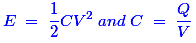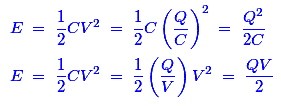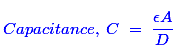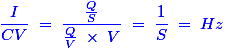# MCQs on Electric Circuits

##### Page 30 of 63. Go to page 1 2 3 4 5 6 7 8 9 10 11 12 13 14 15 16 17 18 19 20 21 22 23 24 25 26 27 28 29 30 31 32 33 34 35 36 37 38 39 40 41 42 43 44 45 46 47 48 49 50 51 52 53 54 55 56 57 58 59 60 61 62 63
01․ The energy stored by a capacitor is given by
1/2 CV2.
Q2 / 2C.
1/2 QV.
all of them.

We know that the basic expression of energy storage by a capacitor is,If we apply this relationship in the expression of energy, we can get the following expression related with that expression.Hence, all of the above expressions are same.

02․ For making a capacitor, it is better to select a dielectric having
low permittivity.
high permittivity.
permittivity same as that of air.
permittivity slightly more than of air.

In order to maximize the charge that a capacitor can hold, the dielectric material needs to have a high permittivity as possible.

03․ The capacitance of a parallel plate capacitor can be varied by
changing the overlapping area of the plates only.
changing the distance between the plates only.
changing the dielectric medium only.
all of the above.

The capacitance for any pair of separated conductors can be found with the below-given expressionWhere, ε is Permittivity of dielectric, A is Area of the plates, D is Distance between the plates. Hence, all the above parameters can vary the value of capacitance.

04․ The power dissipated in a pure capacitor is
zero.
proportional to applied voltage.
proportional to the value of capacitance.
both (B) and (C) above.

In the pure capacitor, phase angle between the current and voltage is exactly 90o. We know that the expression of power is P = V Icosθ. Here, θ  = 90o. Hence, cos90o = 0. So, the power dissipated in a pure capacitor is zero.

05․ If the dielectric of a capacitor is replaced by conducting material
the capacitance value of the plate will decrease.
the capacitor can store infinite charge.
the plate will get short circuited.
the capacitor will get heated up due to eddy currents.

The dielectric material is placed in between the two metal plates. If we replace the dielectric material by a conducting metal, then it leads to make a direct short circuit between the metal plates.

06․ If C be the capacitance, V be the potential difference and I be the current, then I / CV will have the unit of
time.
power.
frequency.
reactive power.

We know that one amp is one coulomb per second and one farad is one coulomb per volt. If we apply these units of current and capacitance in the given expression, we will get (1/Second).Hence, the unit of (I/CV) is frequency.

07․ A variable capacitance is one whose capacitance
changes with temperature.
changes with time.
can be changed manually or by mechanical force.
changes with voltage.

There is a knob to change the capacitance value that done by applying the manual mechanical force. A variable capacitor stores the charge in the same fashion like normal capacitor and it can be adjusted as many times as desired to store different amounts of electricity by adjusting the knob.

08․ A capacitor having a capacitance of 40 μF is connected across 250 V DC source. The charging current will be least
when capacitor is fully charged.
when capacitor is half charged.
when capacitor is almost 25% charged.
initially.

Charging in a capacitor that creates a charge imbalance between the two plates and it creates a reverse voltage that stops the charging process. As a result, when a capacitor is charged, current stops flowing and it becomes an open circuit. That is why the current is least when capacitor is fully charged.

09․ Internal heating of a capacitor is usually attributed to
electron movement.
leakage resistance.
dielectric charge.
plate vibration.

A real capacitor has a leakage resistance internally. It can be said as a large resistance in parallel with capacitor. While the leakage current flowing in it, and then I2R loss is produced due to that leakage resistance. Hence, the internal loss happens due to the leakage power loss inside the capacitor.

10․ In a capacitor, the electrical energy is stored in
magnetic field.
electric field.
both ‘electric field’ and magnetic field’.
none of above.

The energy stored in the capacitor is stored as an electric field and the interesting things is the capacitor as a device which either stores energy in, or extracts energy from, an electric field.

<<<2829303132>>>